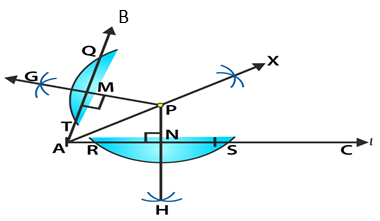# RD Sharma Solutions for Class 6 Maths Chapter 19: Geometrical Constructions Exercise 19.2

The students get familiar with the various Geometrical Constructions by solving problems using solutions prepared by experts at BYJU’S. This exercise contains questions based on constructions using the ruler and set squares. The solutions are mainly prepared with the aim of helping students perform well in the exam and obtain a good academic score. Students who aim to score good marks in Mathematics can use RD Sharma Solutions Class 6 Maths Chapter 19 Geometrical Constructions Exercise 19.2 PDF which are available here for free download.

## RD Sharma Solutions for Class 6 Maths Chapter 19: Geometrical Constructions Exercise 19.2 Download PDF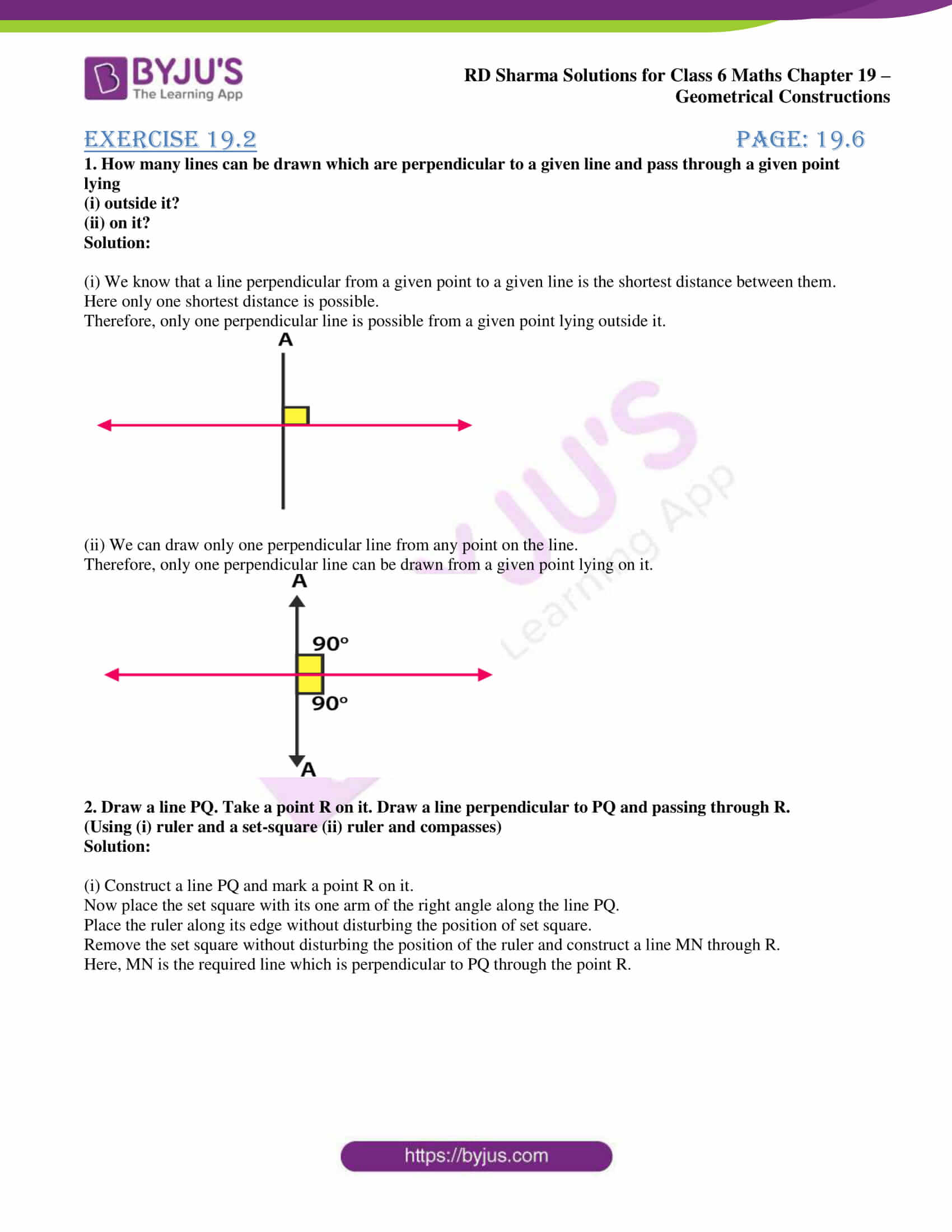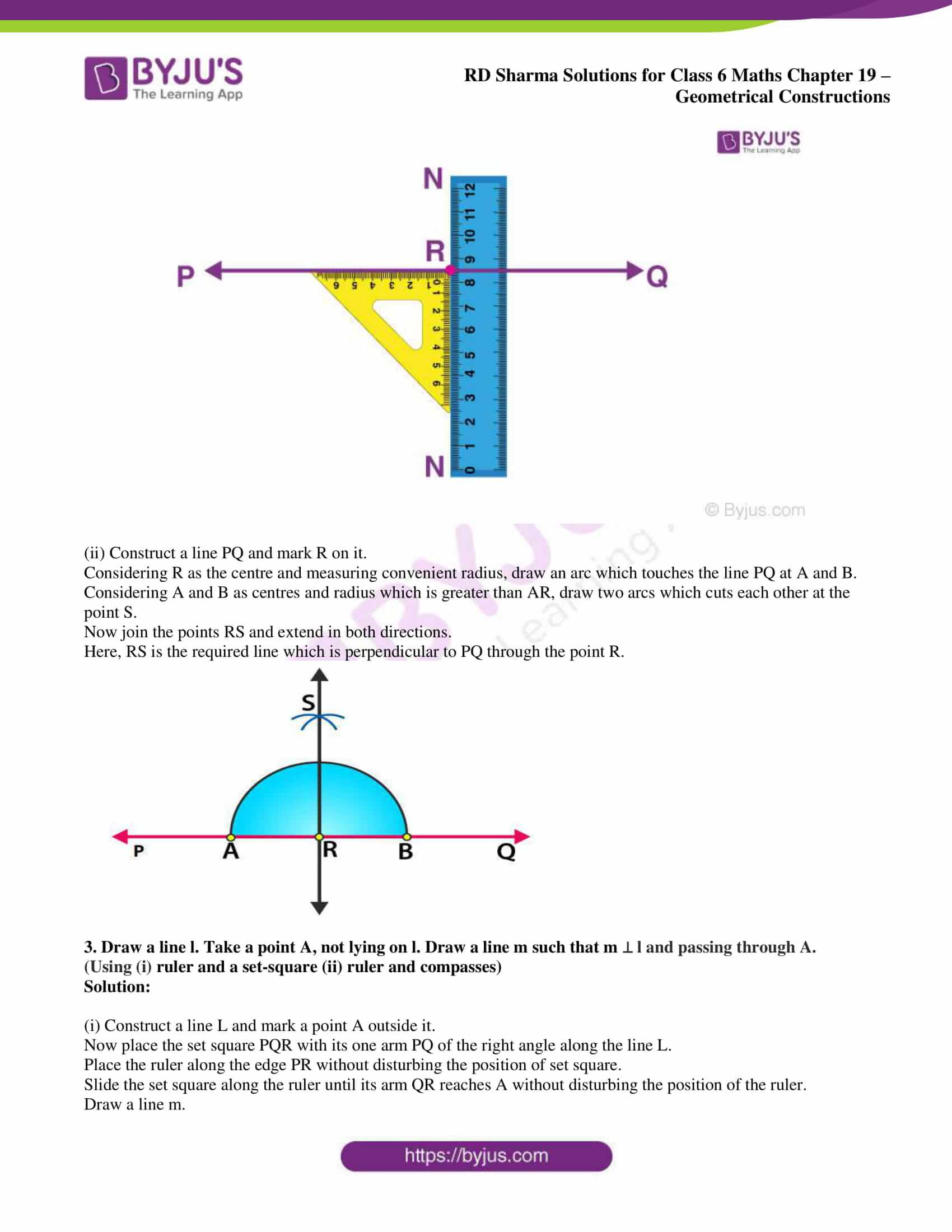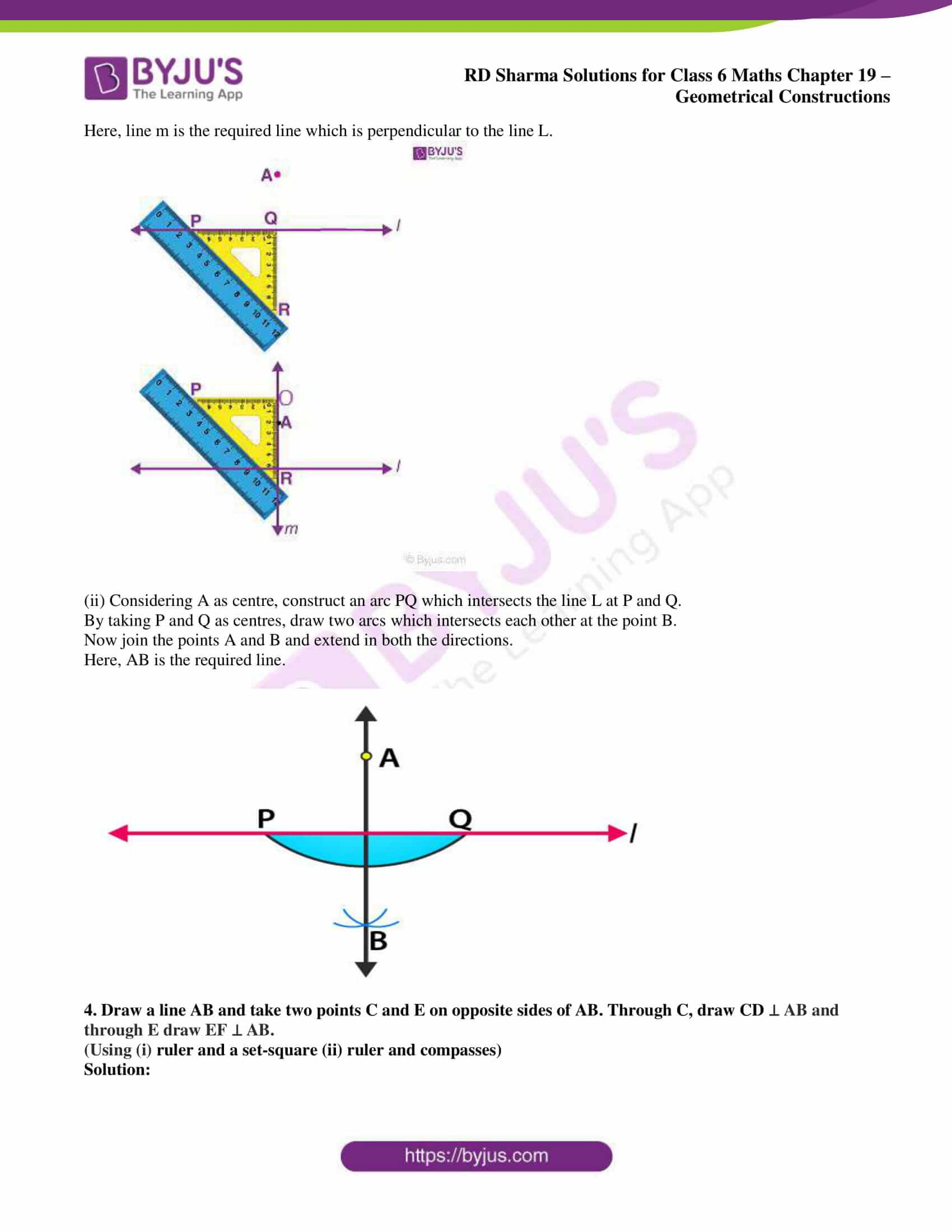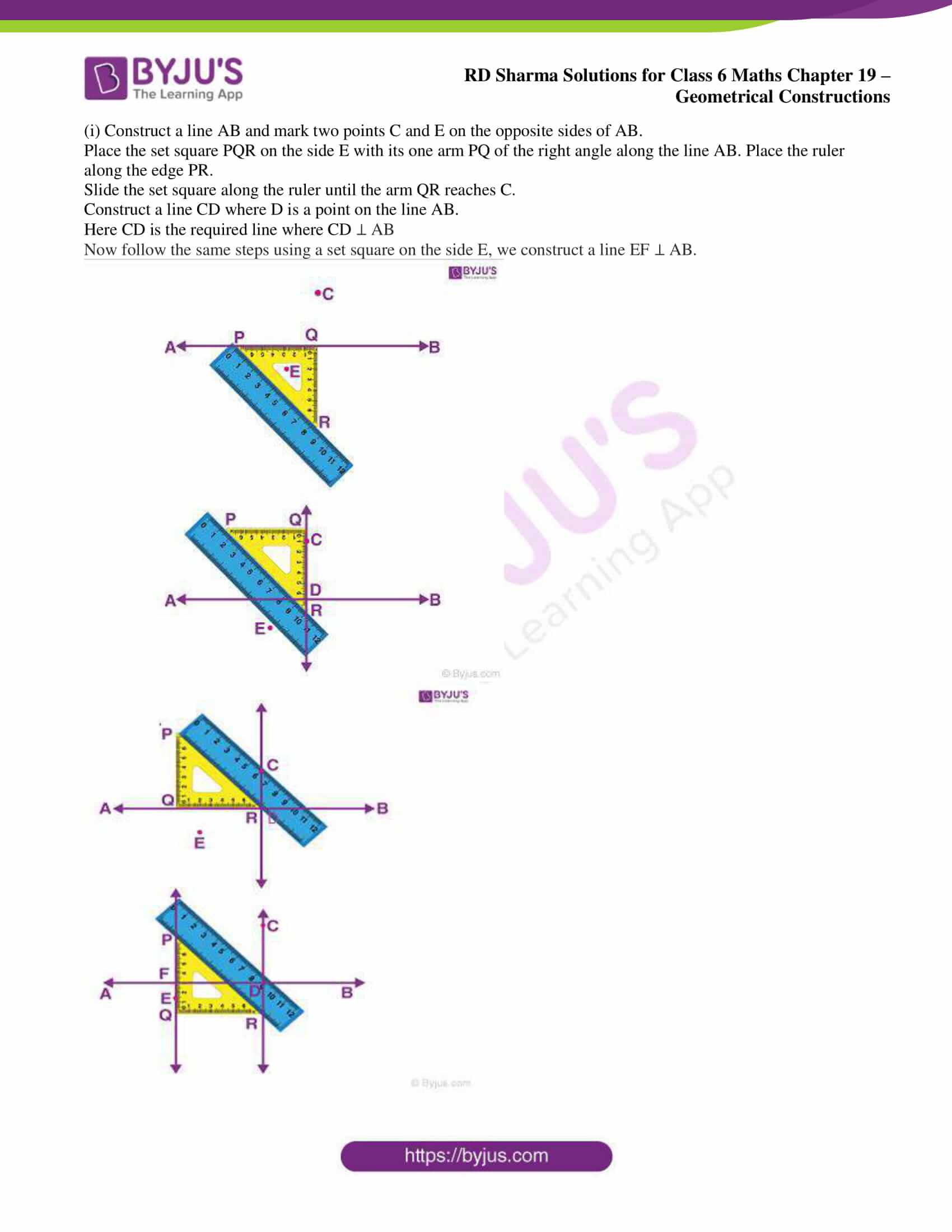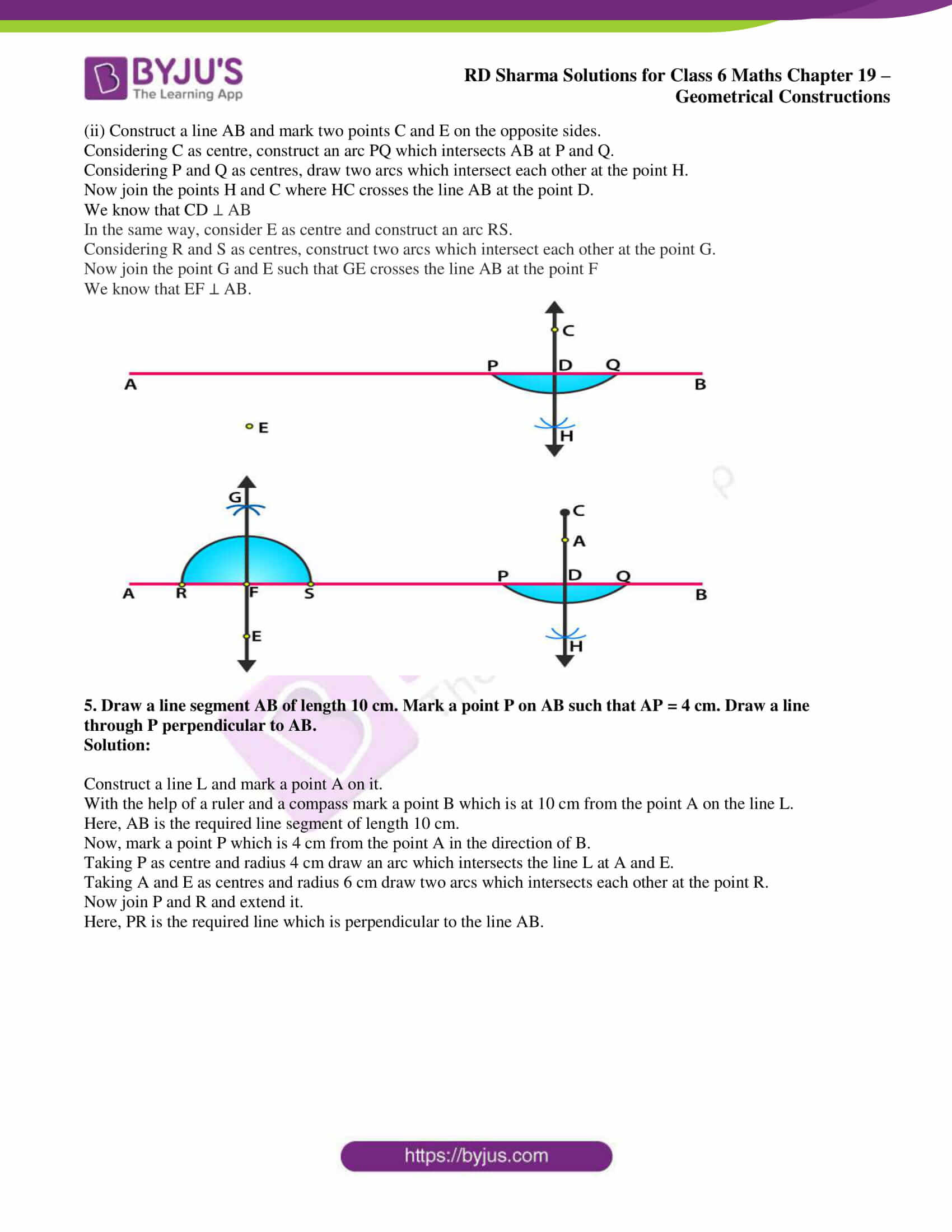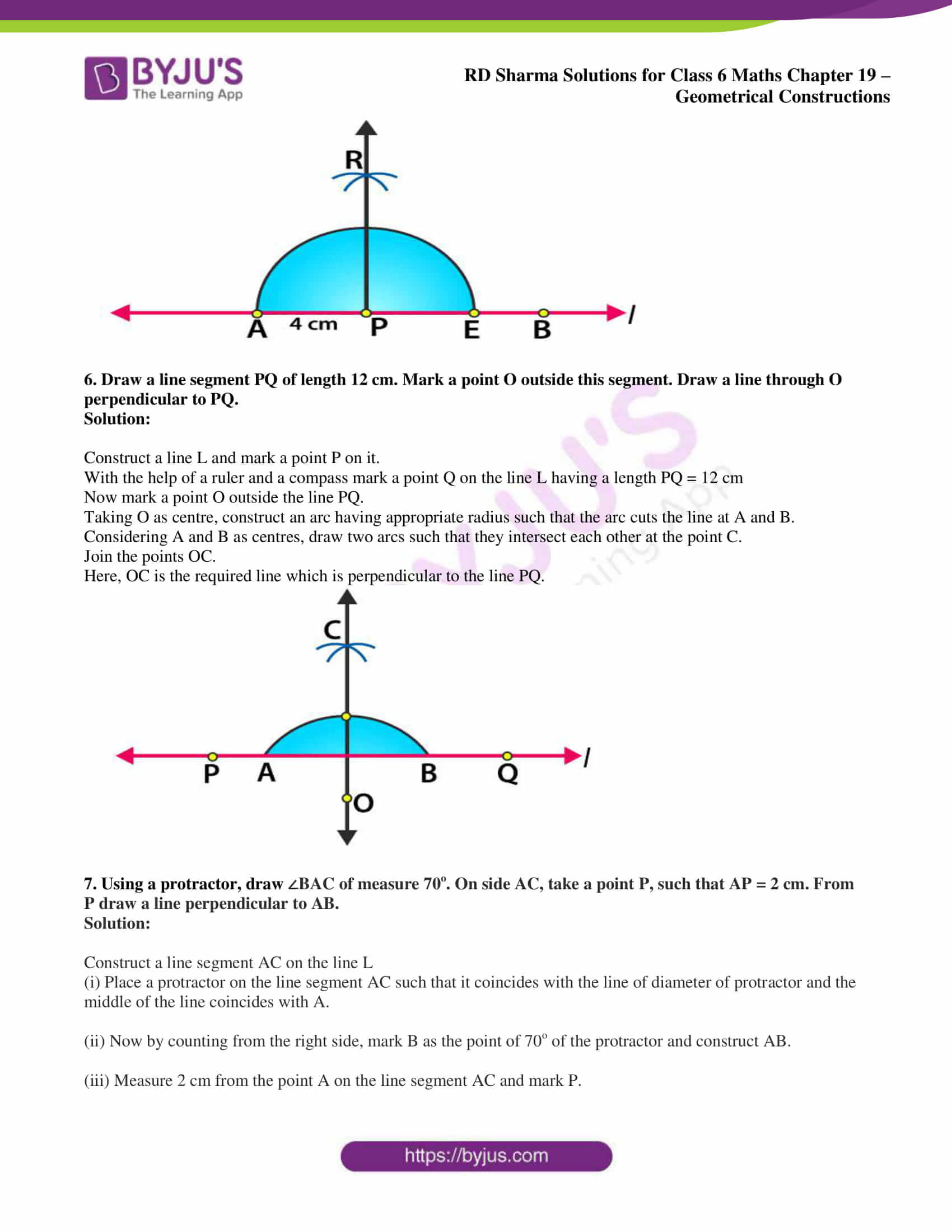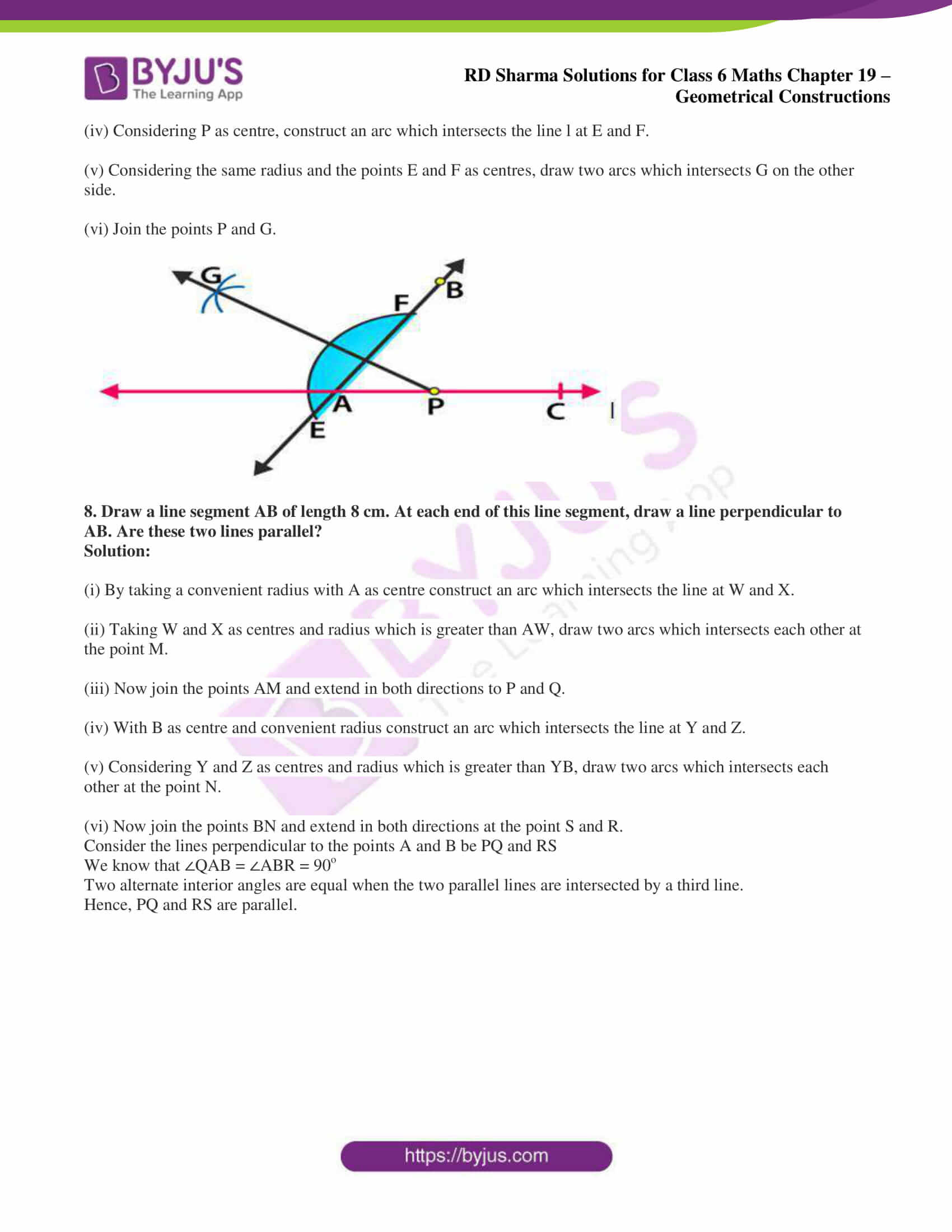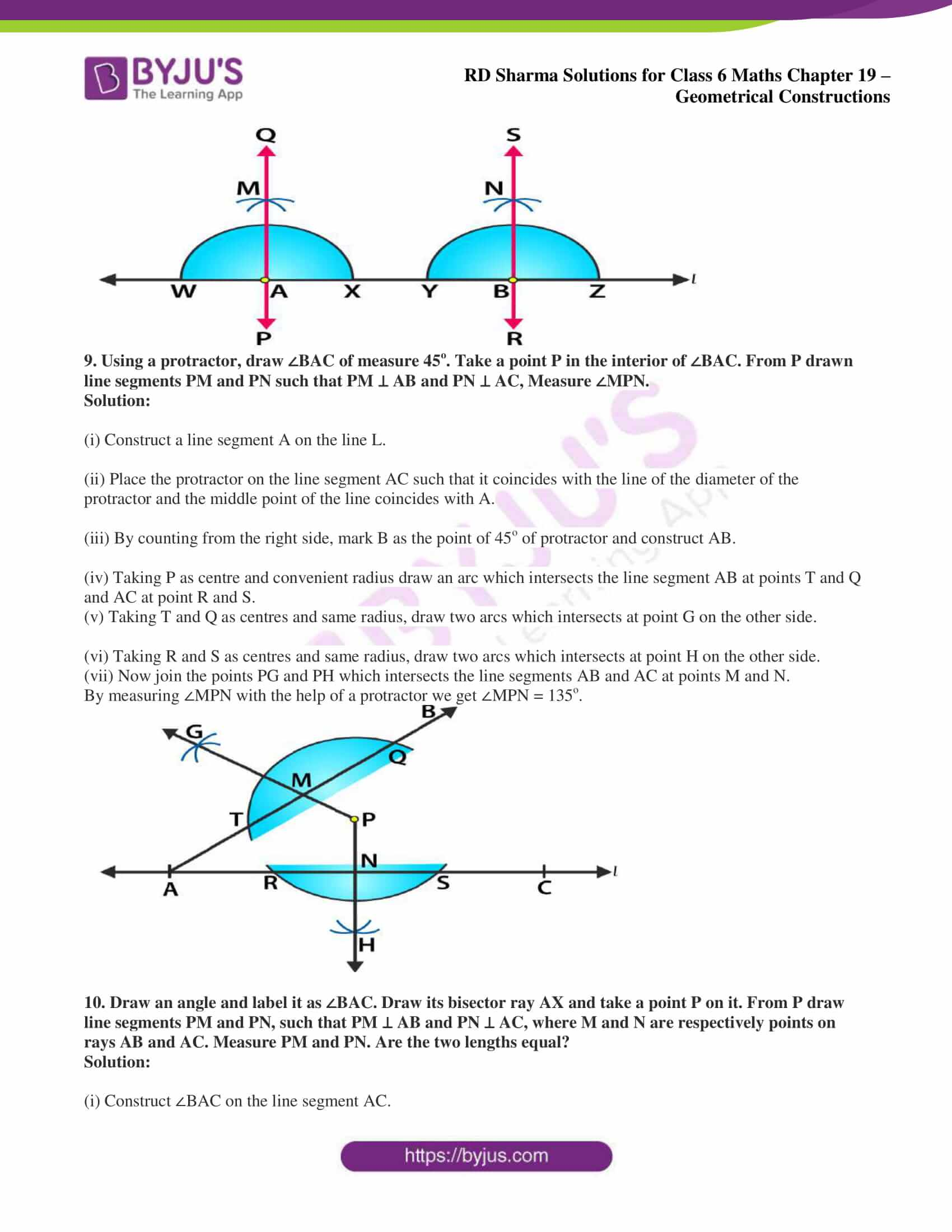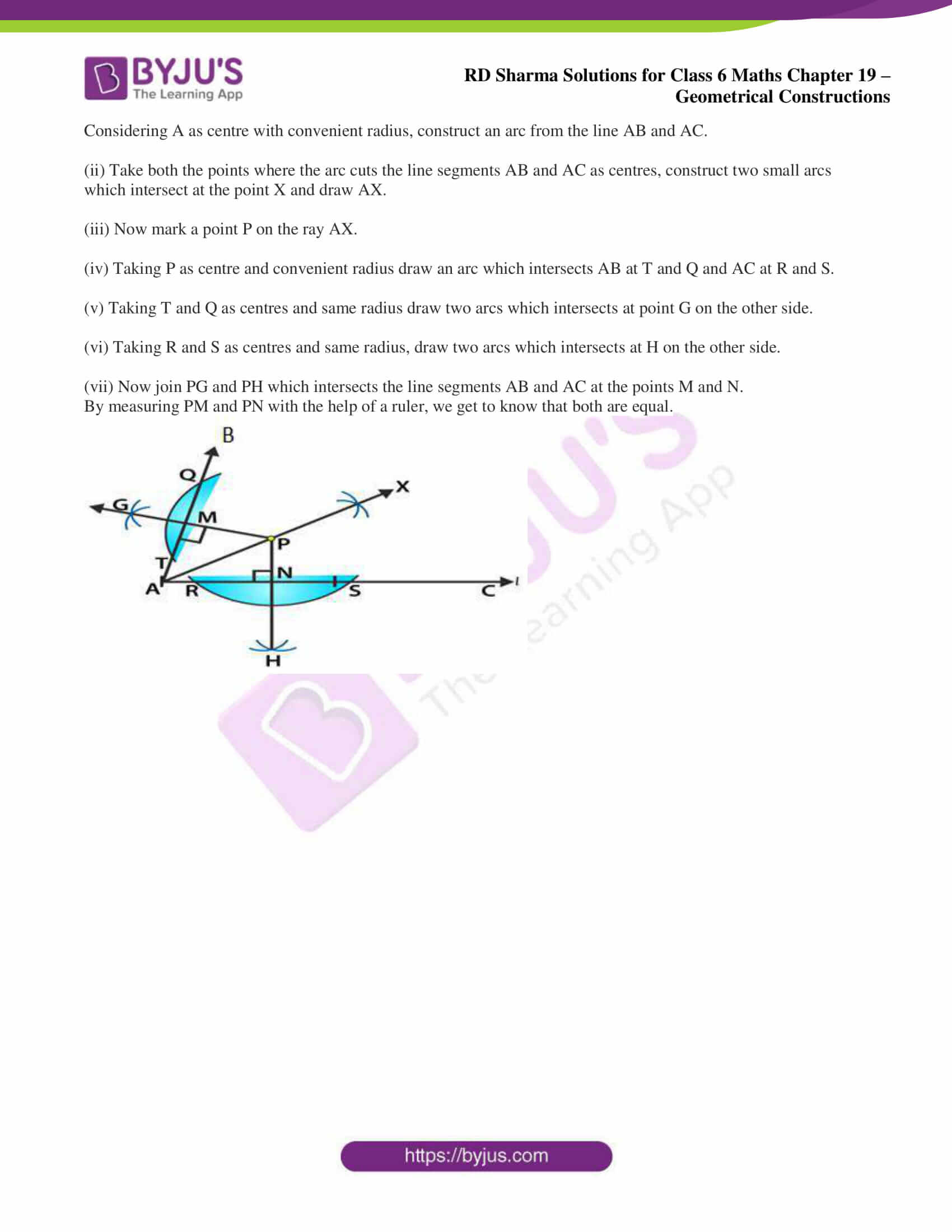### Exercise 19.2 page: 19.6

1. How many lines can be drawn which are perpendicular to a given line and pass through a given point lying

(i) outside it?

(ii) on it?

Solution:

(i) We know that a line perpendicular from a given point to a given line is the shortest distance between them.

Here only one shortest distance is possible.

Therefore, only one perpendicular line is possible from a given point lying outside it.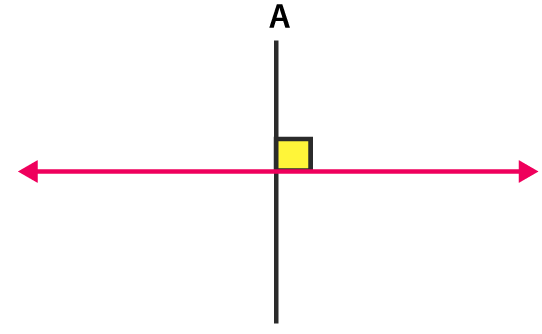(ii) We can draw only one perpendicular line from any point on the line.

Therefore, only one perpendicular line can be drawn from a given point lying on it.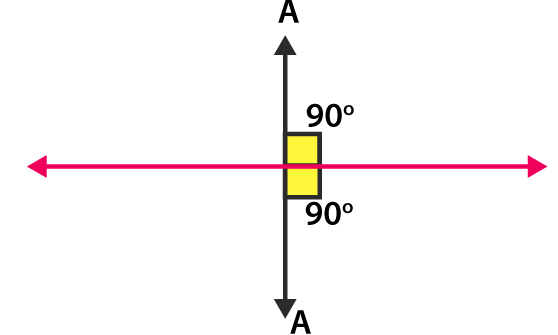2. Draw a line PQ. Take a point R on it. Draw a line perpendicular to PQ and passing through R.

(Using (i) ruler and a set-square (ii) ruler and compasses)

Solution:

(i) Construct a line PQ and mark a point R on it.

Now place the set square with its one arm of the right angle along the line PQ.

Place the ruler along its edge without disturbing the position of set square.

Remove the set square without disturbing the position of the ruler and construct a line MN through R.

Here, MN is the required line which is perpendicular to PQ through the point R.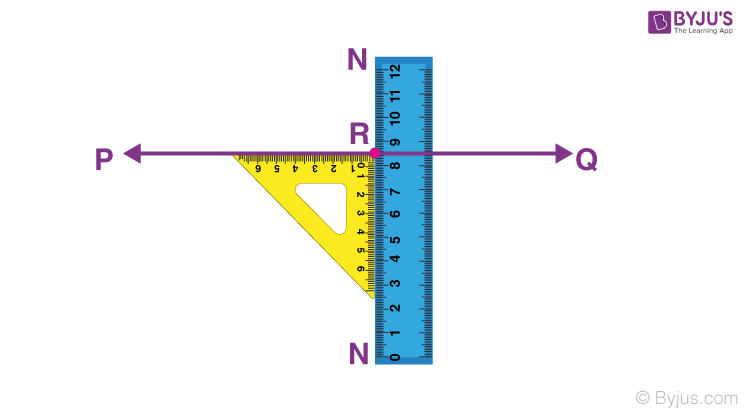(ii) Construct a line PQ and mark R on it.

Considering R as the centre and measuring convenient radius, draw an arc which touches the line PQ at A and B.

Considering A and B as centres and radius which is greater than AR, draw two arcs which cuts each other at the point S.

Now join the points RS and extend in both directions.

Here, RS is the required line which is perpendicular to PQ through the point R.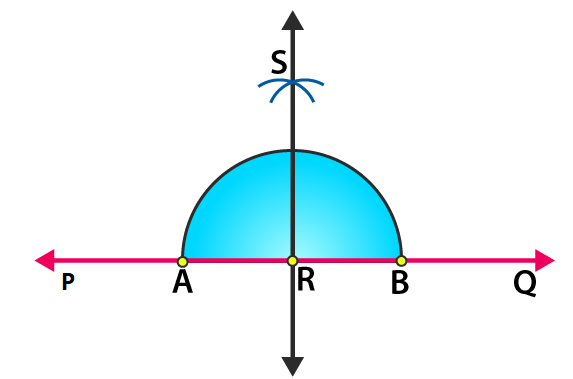3. Draw a line l. Take a point A, not lying on l. Draw a line m such that m ⊥ l and passing through A.

(Using (i) ruler and a set-square (ii) ruler and compasses)

Solution:

(i) Construct a line L and mark a point A outside it.

Now place the set square PQR with its one arm PQ of the right angle along the line L.

Place the ruler along the edge PR without disturbing the position of set square.

Slide the set square along the ruler until its arm QR reaches A without disturbing the position of the ruler.

Draw a line m.

Here, line m is the required line which is perpendicular to the line L.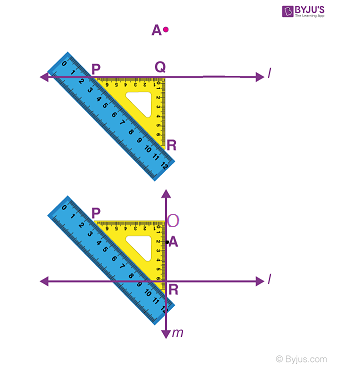(ii) Considering A as centre, construct an arc PQ which intersects the line L at P and Q.

By taking P and Q as centres, draw two arcs which intersects each other at the point B.

Now join the points A and B and extend in both the directions.

Here, AB is the required line.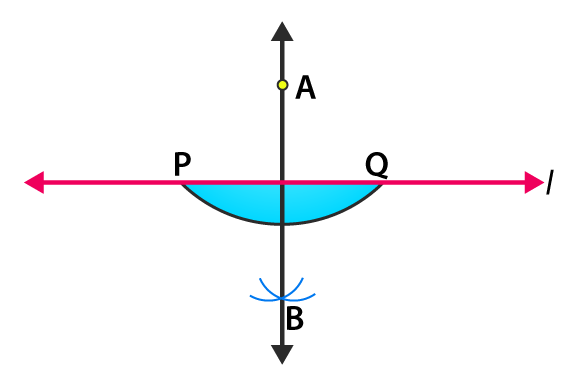4. Draw a line AB and take two points C and E on opposite sides of AB. Through C, draw CD ⊥ AB and through E draw EF ⊥ AB.

(Using (i) ruler and a set-square (ii) ruler and compasses)

Solution:

(i) Construct a line AB and mark two points C and E on the opposite sides of AB.

Place the set square PQR on the side E with its one arm PQ of the right angle along the line AB. Place the ruler along the edge PR.

Slide the set square along the ruler until the arm QR reaches C.

Construct a line CD where D is a point on the line AB.

Here CD is the required line where CD ⊥ AB

Now follow the same steps using a set square on the side E, we construct a line EF ⊥ AB.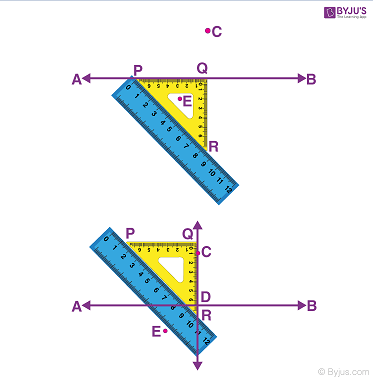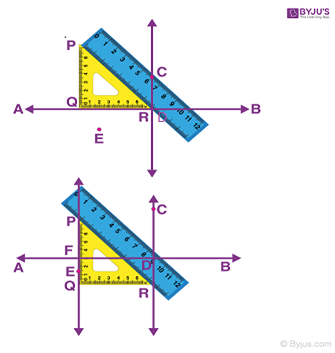(ii) Construct a line AB and mark two points C and E on the opposite sides.

Considering C as centre, construct an arc PQ which intersects AB at P and Q.

Considering P and Q as centres, draw two arcs which intersect each other at the point H.

Now join the points H and C where HC crosses the line AB at the point D.

We know that CD ⊥ AB

In the same way, consider E as centre and construct an arc RS.

Considering R and S as centres, construct two arcs which intersect each other at the point G.

Now join the point G and E such that GE crosses the line AB at the point F

We know that EF ⊥ AB.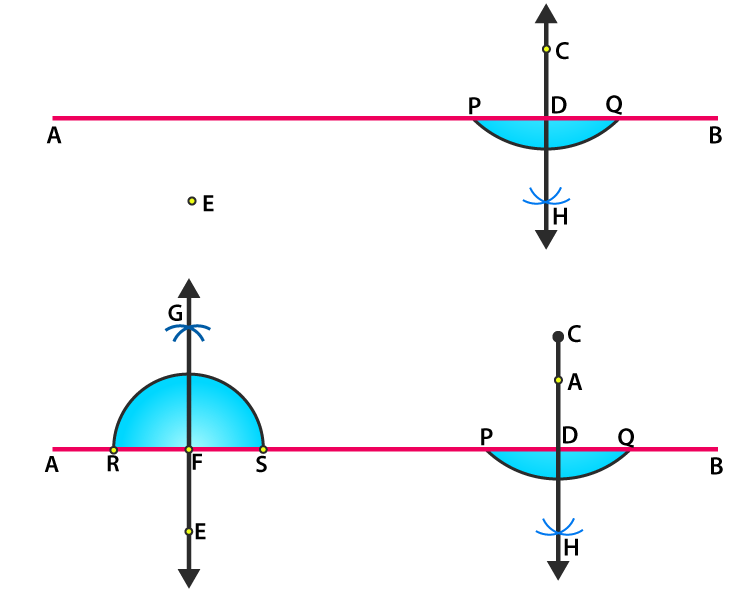5. Draw a line segment AB of length 10 cm. Mark a point P on AB such that AP = 4 cm. Draw a line through P perpendicular to AB.

Solution:

Construct a line L and mark a point A on it.

With the help of a ruler and a compass mark a point B which is at 10 cm from the point A on the line L.

Here, AB is the required line segment of length 10 cm.

Now, mark a point P which is 4 cm from the point A in the direction of B.

Taking P as centre and radius 4 cm draw an arc which intersects the line L at A and E.

Taking A and E as centres and radius 6 cm draw two arcs which intersects each other at the point R.

Now join P and R and extend it.

Here, PR is the required line which is perpendicular to the line AB.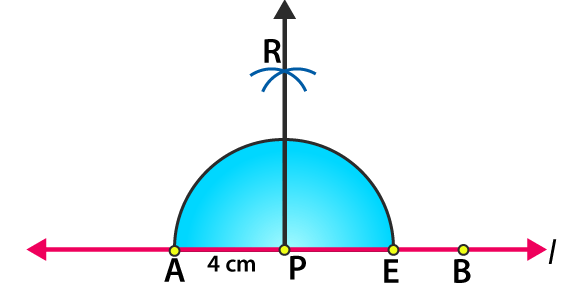6. Draw a line segment PQ of length 12 cm. Mark a point O outside this segment. Draw a line through O perpendicular to PQ.

Solution:

Construct a line L and mark a point P on it.

With the help of a ruler and a compass mark a point Q on the line L having a length PQ = 12 cm

Now mark a point O outside the line PQ.

Taking O as centre, construct an arc having appropriate radius such that the arc cuts the line at A and B.

Considering A and B as centres, draw two arcs such that they intersect each other at the point C.

Join the points OC.

Here, OC is the required line which is perpendicular to the line PQ.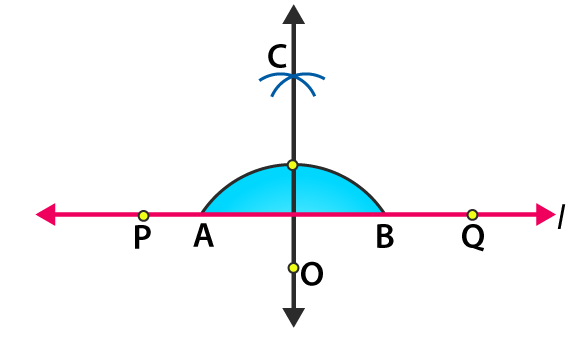7. Using a protractor, draw ∠BAC of measure 70o. On side AC, take a point P, such that AP = 2 cm. From P draw a line perpendicular to AB.

Solution:

Construct a line segment AC on the line L

(i) Place a protractor on the line segment AC such that it coincides with the line of diameter of protractor and the middle of the line coincides with A.

(ii) Now by counting from the right side, mark B as the point of 70o of the protractor and construct AB.

(iii) Measure 2 cm from the point A on the line segment AC and mark P.

(iv) Considering P as centre, construct an arc which intersects the line l at E and F.

(v) Considering the same radius and the points E and F as centres, draw two arcs which intersects G on the other side.

(vi) Join the points P and G.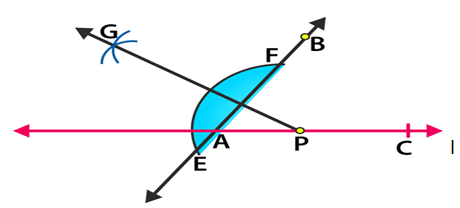8. Draw a line segment AB of length 8 cm. At each end of this line segment, draw a line perpendicular to AB. Are these two lines parallel?

Solution:

(i) By taking a convenient radius with A as centre construct an arc which intersects the line at W and X.

(ii) Taking W and X as centres and radius which is greater than AW, draw two arcs which intersects each other at the point M.

(iii) Now join the points AM and extend in both directions to P and Q.

(iv) With B as centre and convenient radius construct an arc which intersects the line at Y and Z.

(v) Considering Y and Z as centres and radius which is greater than YB, draw two arcs which intersects each other at the point N.

(vi) Now join the points BN and extend in both directions at the point S and R.

Consider the lines perpendicular to the points A and B be PQ and RS

We know that ∠QAB = ∠ABR = 90o

Two alternate interior angles are equal when the two parallel lines are intersected by a third line.

Hence, PQ and RS are parallel.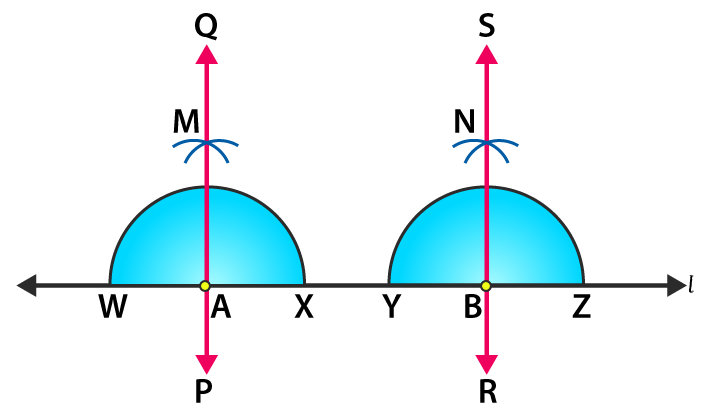9. Using a protractor, draw ∠BAC of measure 45o. Take a point P in the interior of ∠BAC. From P drawn line segments PM and PN such that PM ⊥ AB and PN ⊥ AC, Measure ∠MPN.

Solution:

(i) Construct a line segment A on the line L.

(ii) Place the protractor on the line segment AC such that it coincides with the line of the diameter of the protractor and the middle point of the line coincides with A.

(iii) By counting from the right side, mark B as the point of 45o of protractor and construct AB.

(iv) Taking P as centre and convenient radius draw an arc which intersects the line segment AB at points T and Q and AC at point R and S.

(v) Taking T and Q as centres and same radius, draw two arcs which intersects at point G on the other side.

(vi) Taking R and S as centres and same radius, draw two arcs which intersects at point H on the other side.

(vii) Now join the points PG and PH which intersects the line segments AB and AC at points M and N.

By measuring ∠MPN with the help of a protractor we get ∠MPN = 135o.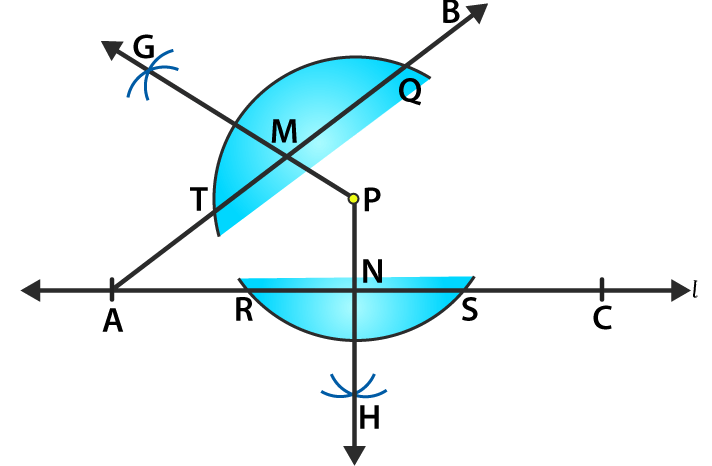10. Draw an angle and label it as ∠BAC. Draw its bisector ray AX and take a point P on it. From P draw line segments PM and PN, such that PM ⊥ AB and PN ⊥ AC, where M and N are respectively points on rays AB and AC. Measure PM and PN. Are the two lengths equal?

Solution:

(i) Construct ∠BAC on the line segment AC.

Considering A as centre with convenient radius, construct an arc from the line AB and AC.

(ii) Take both the points where the arc cuts the line segments AB and AC as centres, construct two small arcs which intersect at the point X and draw AX.

(iii) Now mark a point P on the ray AX.

(iv) Taking P as centre and convenient radius draw an arc which intersects AB at T and Q and AC at R and S.

(v) Taking T and Q as centres and same radius draw two arcs which intersects at point G on the other side.

(vi) Taking R and S as centres and same radius, draw two arcs which intersects at H on the other side.

(vii) Now join PG and PH which intersects the line segments AB and AC at the points M and N.

By measuring PM and PN with the help of a ruler, we get to know that both are equal.